# Code with correction of arithmetical errors

code for correction of arithmetic errors, code that corrects arithmetic errors, arithmetic code

A code intended for the control of the functioning of an adder. When adding numbers represented in the binary number system, a single slip in the functioning of the adder leads, as a rule, to a change in the result by some power of 2. In this connection, a (single) arithmetic error in the ring of integersis defined as a transformation of a numberto a number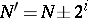,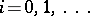. The function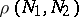on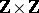, defined as the minimum number of arithmetic errors takingto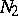, is a metric. An arbitrary finite subset (a code)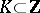is characterized by its minimum distancewhere the minimum is taken over all distinct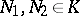. The sets ofor fewer arithmetic errors transform a numberinto a metric ball of radiusand centre. Therefore if the metric balls of radiusdescribed around any two numbers of a code do not intersect (that is, if the minimum distance of the code is greater than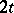), then the code is said to be a code that correctsarithmetic errors. If the metric ball of radiusdescribed around any number of the code contains no other numbers of the code (that is, if the minimum distance of the code is greater than), then the code is called a code that detectsarithmetic errors. The metrichas the following alternative description:whereis the (arithmetic) weight of, i.e. the smallest possible number of non-zero coefficients in the representations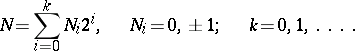(*)

For eachthere is a unique representation (*) on which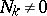,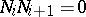,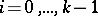, and the number of non-zero coefficients in the representation is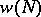. For example,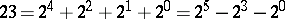and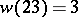. Since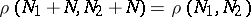for any, one restricts attention to codes that do not contain negative numbers. By the length of a codeone means the quantity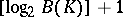, where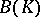is the largest number of the code. There corresponds to an arbitrary numberof a code of lengtha unique word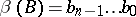over the alphabet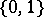such that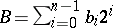. If the codecorrectsarithmetic errors, then the codecorrectserrors (of substitution type) by virtue of the fact that, where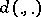is the Hamming metric (see Error-correcting code). In the study of problems of control of an adder one usually considers an encoding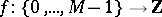(see Coding and decoding) such thatfor(which is equivalent to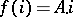, where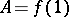). In this case, the coding operation consists in the multiplication of the number to be encoded by, while the detection of errors in the functioning of the adder consists in verifying that the result of the addition is divisible by. The code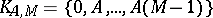is called an-code. The minimum distance of an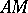-code is the minimum of the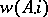,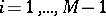. For example, the minimum distance of a-code is equal to 2; the code detects single arithmetic errors and is often applied in computers (in the form of a control modulo 3). The best studied are (cyclic and negacyclic)-codes with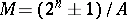; they are characterized by the property that whenever the number,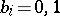, belongs to the code, then so does the number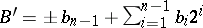. Such codes, called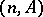-codes, can be conveniently regarded as subsets of the metric space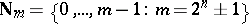, with metric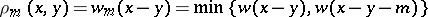. The minimum distances of an-code in the metricsandare equal. To each-code there is associated the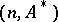-code where; it is called the dual code. If 2 or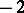are primitive roots modulo a prime number, then the-code corrects single arithmetic errors and is perfect in the metric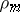, that is, the metric balls of radius 1 described around the numbers of the code are not only pairwise disjoint but also fill out the whole of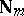. In the dual code, all distances between different numbers of the code are equal to. The results listed above for codes are analogues of results for binary linear cyclic error-correcting codes. Meanwhile, there are no known arithmetic analogues of B.C.H. (Bose–Chaudhury–Hocquenghem)-codes; nor are there any known upper bounds for the size of codes with given length and distance, analogous to the best bound for error-correcting codes. Codes correcting-ary arithmetic errors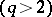possess even more characteristics (a-ary arithmetical error is a transformation of a numberto a number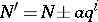,;). Interest in such codes has increased with the development of computing techniques. Thus, in contrast to error-correcting codes, there are no perfect-codes with(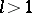) that correct single-ary arithmetic errors; forthere are examples known of such codes. Existence conditions for perfect-codes are of a number-theoretic character and are connected with the study of reciprocity laws in number fields.